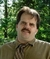## Result of a stringTags:
String
TextBox
Hi, sorry but I have a simple question! I have a string like "2^5+2*5^2-1" in a textbox and I want to have the result of this string in another textbox. But I can't get the answer! Please help!

Thanks. We'll let you know when a new response is added.

## Discuss This Question: 6 Replies

Thanks. We'll let you know when a new response is added.
•One way to do it is by using postfix notation.

See Postfix Evaluation
report
•Looks like a homework question.

Post what you have tried and we can help clear it up.
report
Yes, this is for split-half method to obtain nearest root of a non-linear function like f(x) in university for Numerical Calculations lesson.
It is so odd for me that for obtaining the result of a string in VB we can't do it straight !!!!!! it is so simple !!!
I have the code of the program in matlab but I want to change it to VB.
```function [c language=",k,err"][/c]=tansif(f,a,b,e)
% 'c' value of root
% 'k' number of loop
% 'err' exact value of error
% 'f' function in 'inline' input
% [a,b] given intervals, and e is the value of error
ezplot(f,[a,b])
hold on
ezplot('0',[a,b])
if nargin<4
e=0.001;
end
format long
format compact
k=0;
if f(a)*f(b)<0
while abs(a-b)>e
c=(a+b)/2;
if f(a)*f(c)<0
disp([a,b,c]);
b=c;
k=k+1;
else
disp([a,b,c]);
a=c;
k=k+1;
end
end
err=abs(a-b);
else
disp('given function has no root in this intervals');
end
plot(c,f(c),'.r')```
of course the plot section is not important.
report
• great !!! so many experts !
report
• I have the code of the program in matlab but I want to change it to VB.

And what problem are you having in doing so? (Are you asking us to do it for you?)
report
• Nope, I just ask to help find out how to get a string format function value !! like the first post. They asked for more detail and I sent the code ! It's enough to help me on first post.
report